# 123 Results

View
Selected filters:
• Mathematics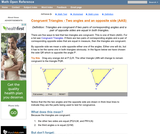Rating

An interactive applet and associated web page that shows how triangles that have two angles and a non-included side the same must be congruent. The applet shows two triangles, one of which can be reshaped by dragging any vertex. The other changes to remain congruent to it and the two angles and non-included side are outlined in bold to show they are the same measure and are the elements being used to prove congruence. The web page describes all this and has links to other related pages. Applet can be enlarged to full screen size for use with a classroom projector. This resource is a component of the Math Open Reference Interactive Geometry textbook project at http://www.mathopenref.com.

Material Type:
Simulation
Provider:
Math Open Reference
Author:
John Page
02/16/2011Unrestricted Use
CC BY
Rating

This task examines the ways in which the plane can be covered by regular polygons in a very strict arrangement called a regular tessellation. These tessellations are studied here using algebra, which enters the picture via the formula for the measure of the interior angles of a regular polygon (which should therefore be introduced or reviewed before beginning the task). The goal of the task is to use algebra in order to understand which tessellations of the plane with regular polygons are possible.

Material Type:
Activity/Lab
Provider:
Illustrative Mathematics
Provider Set:
Illustrative Mathematics
Author:
Illustrative Mathematics
01/21/2013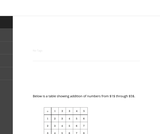Unrestricted Use
CC BY
Rating

The purpose of this task is to study some patterns in a small addition table. Each pattern identified persists for a larger table and if more time is available for this activity students should be encouraged to explore these patterns in larger tables.

Material Type:
Activity/Lab
Provider:
Illustrative Mathematics
Provider Set:
Illustrative Mathematics
Author:
Illustrative Mathematics
08/15/2012Conditional Remix & Share Permitted
CC BY-NC-SA
Rating

In this activity, learners use a hand-made protractor to measure angles they find in playground equipment. Learners will observe that angle measurements do not change with distance, because they are distance invariant, or constant. Note: The "Pocket Protractor" activity should be done ahead as a separate activity (see related resource), but a standard protractor can be used as a substitute.

Material Type:
Activity/Lab
Provider:
Exploratorium
Author:
Exploratorium
Gordon and Betty Moore Foundation
National Science Foundation
The Exploratorium
12/07/2010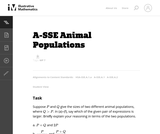Unrestricted Use
CC BY
Rating

In this task students have to interpret expressions involving two variables in the context of a real world situation. All given expressions can be interpreted as quantities that one might study when looking at two animal populations.

نوع المادة:
Activity/Lab
Provider:
Illustrative Mathematics
Provider Set:
Illustrative Mathematics
المؤلف:
Illustrative Mathematics
05/01/2012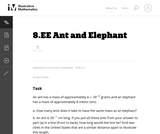Unrestricted Use
CC BY
Rating

In this problem students are comparing a very small quantity with a very large quantity using the metric system. The metric system is especially convenient when comparing measurements using scientific notations since different units within the system are related by powers of ten.

Material Type:
Activity/Lab
Provider:
Illustrative Mathematics
Provider Set:
Illustrative Mathematics
Author:
Illustrative Mathematics
05/01/2012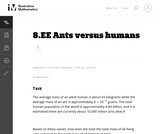Unrestricted Use
CC BY
Rating

This task requires students to work with very large and small values expressed both in scientific notation and in decimal notation (standard form). In addition, students need to convert units of mass.

Material Type:
Activity/Lab
Provider:
Illustrative Mathematics
Provider Set:
Illustrative Mathematics
Author:
Illustrative Mathematics
08/21/2012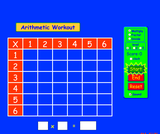Unrestricted Use
CC BY
Rating

Brush up on your multiplication, division, and factoring skills with this interactive multiplication chart. Three levels and timed or untimed options are available.

Material Type:
Simulation
Provider:
Provider Set:
PhET Interactive Simulations
Author:
Michael Dubson
11/05/2011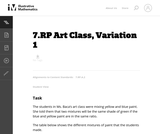Unrestricted Use
CC BY
Rating

This this task about mixing paint requires students to graph ratios on a coordinate plane. It is a standard language in ratio problem.

Material Type:
Activity/Lab
Provider:
Illustrative Mathematics
Provider Set:
Illustrative Mathematics
Author:
Illustrative Mathematics
05/01/2012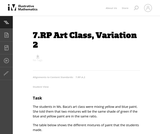Unrestricted Use
CC BY
Rating

This this task about mixing paint requires students to graph ratios on a coordinate plane. It is a standard language in ratio problem.

Material Type:
Activity/Lab
Provider:
Illustrative Mathematics
Provider Set:
Illustrative Mathematics
Author:
Illustrative Mathematics
05/01/2012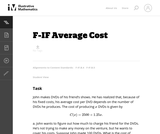Unrestricted Use
CC BY
Rating

In this real world problem students solve questions based on the relationship between production costs and price.

Material Type:
Activity/Lab
Provider:
Illustrative Mathematics
Provider Set:
Illustrative Mathematics
Author:
Illustrative Mathematics
05/01/2012Unrestricted Use
CC BY
Rating

This task provides a real world context for interpreting and solving exponential equations. There are two solutions provided for part (a). The first solution demonstrates how to deduce the conclusion by thinking in terms of the functions and their rates of change. The second approach illustrates a rigorous algebraic demonstration that the two populations can never be equal.

Material Type:
Activity/Lab
Provider:
Illustrative Mathematics
Provider Set:
Illustrative Mathematics
Author:
Illustrative Mathematics
05/01/2012Unrestricted Use
CC BY
Rating

نوع المادة:
Activity/Lab
Provider:
Illustrative Mathematics
Provider Set:
Illustrative Mathematics
المؤلف:
Illustrative Mathematics
05/01/2012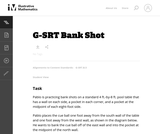Unrestricted Use
CC BY
Rating

This task asks students to use similarity to solve a problem in a context that will be familiar to many, though most students are accustomed to using intuition rather than geometric reasoning to set up the shot.

Material Type:
Activity/Lab
Provider:
Illustrative Mathematics
Provider Set:
Illustrative Mathematics
Author:
Illustrative Mathematics
08/21/2012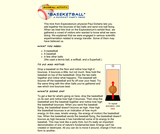Conditional Remix & Share Permitted
CC BY-NC-SA
Rating

This trick from Exploratorium physicist Paul Doherty lets you add together the bounces of two balls and send one ball flying. When we tried this trick on the Exploratorium's exhibit floor, we gathered a crowd of visitors who wanted to know what we were doing. We explained that we were engaged in serious scientific experimentation related to energy transfer. Some of them may have believed us. If you'd like to go into the physical calculations of this phenomenam, see the related resource "Bouncing Balls" - it's the same activity but with the math explained.

Material Type:
Activity/Lab
Provider:
Exploratorium
Author:
Paul Doherty
The Exploratorium
11/07/2012Only Sharing Permitted
CC BY-NC-ND
Rating

This activity explores the main algorithms that are used as the basis for searching on computers, using different variations on the game of battleships. This activity demonstrates three search methods for finding information in data: linear searching, binary searching and hashing. It also includes an optional introductory activity as well as a video showing a fun demonstration related to the same content.

نوع المادة:
Activity/Lab
Game
Lesson Plan
محاكاة
Provider:
Computer Science Unplugged
Science and Math Informal Learning Educators (SMILE)
Provider Set:
Computer Science Unplugged
SMILE Pathway: Science and Math Activities in One Search
المؤلف:
Brian Mason Scientific and Technical Trust
Computer Science Unplugged
Ian Witten
Jane McKenzie
Mike Fellows
Tim Bell
01/02/2002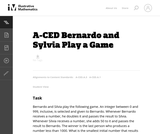Unrestricted Use
CC BY
Rating

This task presents a simple but mathematically interesting game whose solution is a challenging exercise in creating and reasoning with algebraic inequalities. The core of the task involves converting a verbal statement into a mathematical inequality in a context in which the inequality is not obviously presented, and then repeatedly using the inequality to deduce information about the structure of the game.

Material Type:
Activity/Lab
Provider:
Illustrative Mathematics
Provider Set:
Illustrative Mathematics
Author:
Illustrative Mathematics
10/21/2013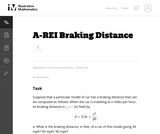Unrestricted Use
CC BY
Rating

This task provides an exploration of a quadratic equation by descriptive, numerical, graphical, and algebraic techniques. Based on its real-world applicability, teachers could use the task as a way to introduce and motivate algebraic techniques like completing the square, en route to a derivation of the quadratic formula.

Material Type:
Activity/Lab
Provider:
Illustrative Mathematics
Provider Set:
Illustrative Mathematics
Author:
Illustrative Mathematics
05/01/2012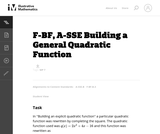Unrestricted Use
CC BY
Rating

This task is for instructional purposes only and builds on ``Building an explicit quadratic function.''

نوع المادة:
Activity/Lab
Provider:
Illustrative Mathematics
Provider Set:
Illustrative Mathematics
المؤلف:
Illustrative Mathematics
08/20/2012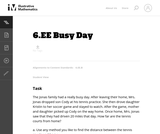Unrestricted Use
CC BY
Rating

This task provides a good entry point for students into representing quantities in contexts with variables and expressions and building equations that reflect the relationships presented in the context.

نوع المادة:
Activity/Lab
Provider:
Illustrative Mathematics
Provider Set:
Illustrative Mathematics
المؤلف:
Illustrative Mathematics